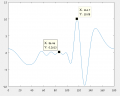# how to shape a formula pattern by optimization

#### yef smith

Joined Aug 2, 2020
606
Hello, I have built a formula which has a plot as shown bellow in the photo and matlab code.
my formula has 8 "a" and 8 "f" coeffients which decide the shape of the plot.
How can i do an optimization so the shape of the plot will be as close as possible to some desired shape?
Is there some practical manual i could read and implement in matlab?Code:
lambda=60;
d=30;
theta=0:0.001:pi;
theta_angle=(theta/pi)*180;
beta=(2*pi)/lambda;
phase=beta*d*sin(pi/6);
ksi=beta*d*cos(theta)+phase;
a1=1;
f1=0;
a2=0.58;
f2=(100.3/180)*3.14;
a3=1.98;
f3=(-107.5/180)*3.14;
a4=3.37;
f4=(-59.4/180)*3.14;
a5=2.72;
f5=(-35.9/180)*3.14;
a6=2.02;
f6=(-33.6/180)*3.14;
a7=1.09;
f7=(-21/180)*3.14;
a8=0.6;
f8=(-53.17/180)*3.14;
e1=a1*exp(1i*(ksi+f1));
e2=a2*exp(1i*(2*ksi+f2));
e3=a3*exp(1i*(3*ksi+f3));
e4=a4*exp(1i*(4*ksi+f4));
e5=a5*exp(1i*(5*ksi+f5));
e6=a6*exp(1i*(6*ksi+f6));
e7=a7*exp(1i*(7*ksi+f7));
e8=a8*exp(1i*(8*ksi+f8));
total=e1+e2+e3+e4+e5+e6+e7+e8;
plot(theta_angle,total);

#### Deleted member 115935

Joined Dec 31, 1969
0
look up least squares
cubic spline
all ways of fitting a curve

#### Tesla23

Joined May 10, 2009
537
I think you are asking about antenna pattern synthesis. Look it up, familiarise yourself with the work that's out there.